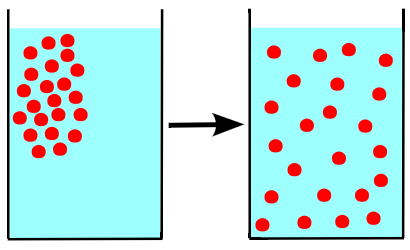## Intermolecular Forces and Solutions

#### Learning Objective

• Recall the two conceptual steps necessary to dissolve a solute and form a solution

#### Key Points

• There are two conceptual steps to form a solution, each corresponding to one of the two opposing forces that dictate solubility.
• The first conceptual step is dissolution, which corresponds to the force of the solvent-solvent and solute-solute intermolecular attractions that needs to be broken down.
• The second conceptual step is solvation, which corresponds to the force of the solute-solvent intermolecular attraction that needs to be formed in order to form a solution.
• Many intermolecular forces can contribute to solvation, including hydrogen bonding, dipole-dipole forces, Van Der Waals forces, and ion-dipole interactions.

#### Term

• intermolecular forcesattractive and repulsive forces between molecules

The strength of the intermolecular forces between solutes and solvents determines the solubility of a given solute in a given solvent. In order to form a solution, the solute must be surrounded, or solvated, by the solvent. Solutes successfully dissolve into solvents when solute-solvent bonds are stronger than either solute-solute bonds or solvent-solvent bonds.

Qualitatively, one can determine the solubility of a solute in a solvent by using the rule “like dissolves like”. In general, solutes whose polarity matches that of the solvent will generally be soluble. For example, table salt (NaCl) dissolves easily into water (H2O) because both molecules are polar.

## Intermolecular Forces and Their Importance in Solution Formation

There are two conceptual steps to form a solution, each corresponding to one of the two opposing forces that dictate solubility. If the solute is a solid or liquid, it must first be dispersed — that is, its molecular units must be pulled apart. This requires energy, and so this step always works against solution formation (always endothermic, or requires that energy be put into the system).Step 1 of dissolutionMolecules going from an ordered state, such as a solid, to a disordered state require an input of energy.The nature of the solute (X) and solvent (Y) determines whether dissolution is energetically favorable or unfavorable. If the solute binds to other solute (X-X bond) more strongly than the solute binds to the solvent (X-Y bond), then the dissolution is not energetically favorable.

## Step 1: Dissolving Solutes

The nature of the solute (X) and solvent (Y) determines whether dissolution is energetically favorable or unfavorable. If the solute binds to other solute (X-X bond) more strongly than the solute binds to the solvent (X-Y bond), then the dissolution is not energetically favorable.

On the other hand, dissolution is favorable when solute-solvent bonds (X-Y) are stronger than X-X or Y-Y bonds. In this case, the potential energy is lower when the solute and solvent can form bonds. If the X-Y attractions are stronger than the X-X or Y-Y attractions, the dissolution reaction is exothermic and releases energy when the solute and solvent are combined.

Since the dissolution of the solvent (X-X) and solute (Y-Y) is always positive, the determining factor for solution formation is the value of X-Y. Remember that the interactions between X and Y, represented above as X-Y, are classified as intermolecular forces, which are not covalent (bonding) interactions.

## Step 2: Forming a Solution

After dissolution occurs, solvation follows. If solvation releases more energy than is consumed during dissolute, then solution formation is favored and the solute is soluble in the solvent. Many intermolecular forces can contribute to solvation, including hydrogen bonding, dipole-dipole forces, and Van Der Waals forces.

## Ion-Dipole Interactions

Another common example of these forces at work is an ion-dipole interaction, which arises when water solvates ions in solution. This interaction arises most prevalently when strong or weak electrolytes are place in water. Consider the dissolution of table salt (sodium chloride) in water:

$NaCl (s) \rightarrow Na^+(aq)+Cl^-(aq)$

The water molecules form a solvent cage around each Na+ or Cl ion, as implied by the aqueous state symbol (aq) following each of the products. The positive ion, Na+, is surrounded by water molecules that have the negative dipoles of the water, or the oxygen, pointing towards the cation.Solvation of a cation by water.Water molecules (gray/green is hydrogen, orange is oxygen) surround a sodium cation in a solution. Notice the negative dipole or the oxygen molecules are ‘facing’ the Na+.

In this case, the anion Cl is solvated by the positive dipoles of water, which are represented by hyrogen atoms. In general, when ions are present in water, each cation and anion is surrounded by a ‘cage’ of partial negative or partial positive charge, respectively. These interactions explain why most ionic compounds are considered soluble in water, unless specifically labeled otherwise.